# Solved Problems on Acidity and Basicity

| Home | | Organic Chemistry |

## Chapter: Organic Chemistry : Acidity and Basicity

Questions and answers, Solved Problems on Acidity and Basicity - Organic Chemistry : Acidity and BasicityPROBLEMS

3.1. Using the pKa data from Table 3.1, predict the position of the following equilibria and give an estimate of the equilibrium constant:

(a) NH3 + OH  ↔ NH2 + H2O

(b) CH3CO2H + CO3−2  ↔ CH3CO2− + HCO3

(c) RCO2H + R2NH  ↔  RCO2 + R2NH2+

(d)(e) NaF + HCl  ↔ NaCl + HF

(f) ArOH + RNH2   ↔ ArO + RNH3+

(g) RC(O)OR′ + H2SO4  ↔ RC(OH+)OR′ + HSO4

(h) RC(O)CH2C(O)R′ + R3N  ↔(i) RC ≡ C + RCH2OH  ↔  RC ≡ CH + RCH2O

(j) CH3Li + (CH3)2C = CH2 ↔ CH4 + (CH3)2C=CHLi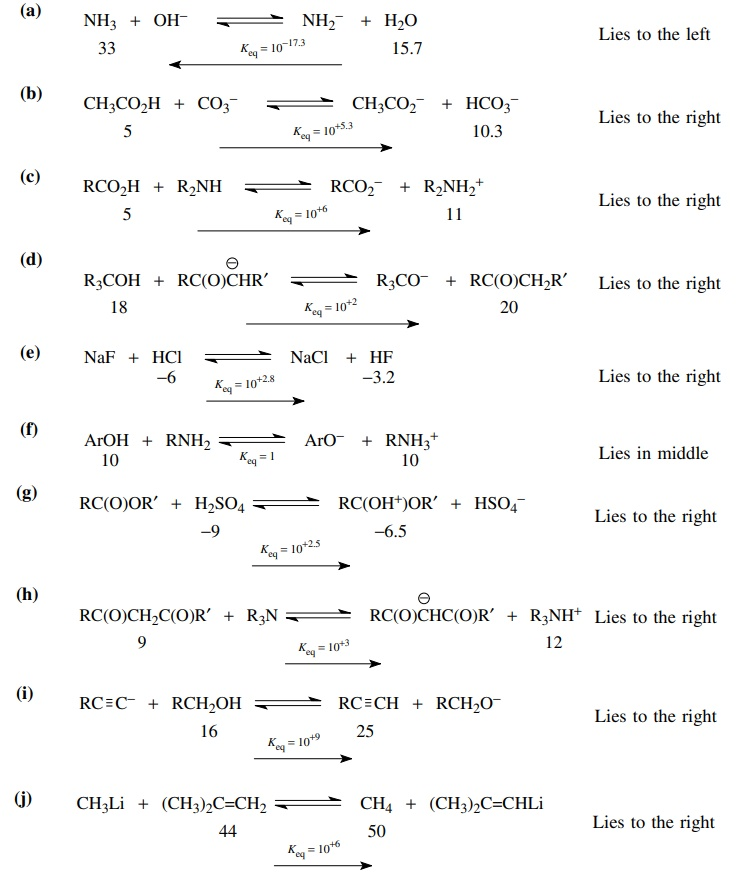3.2. Predict the positions of the following equilibria (left, right, or center) and give an approximate pK value for the equilibrium. You may need to look up or estimate pKa values using structural effects on the pKa values of known compounds. If you do estimate the pKa’s, rationalize your method of estimation.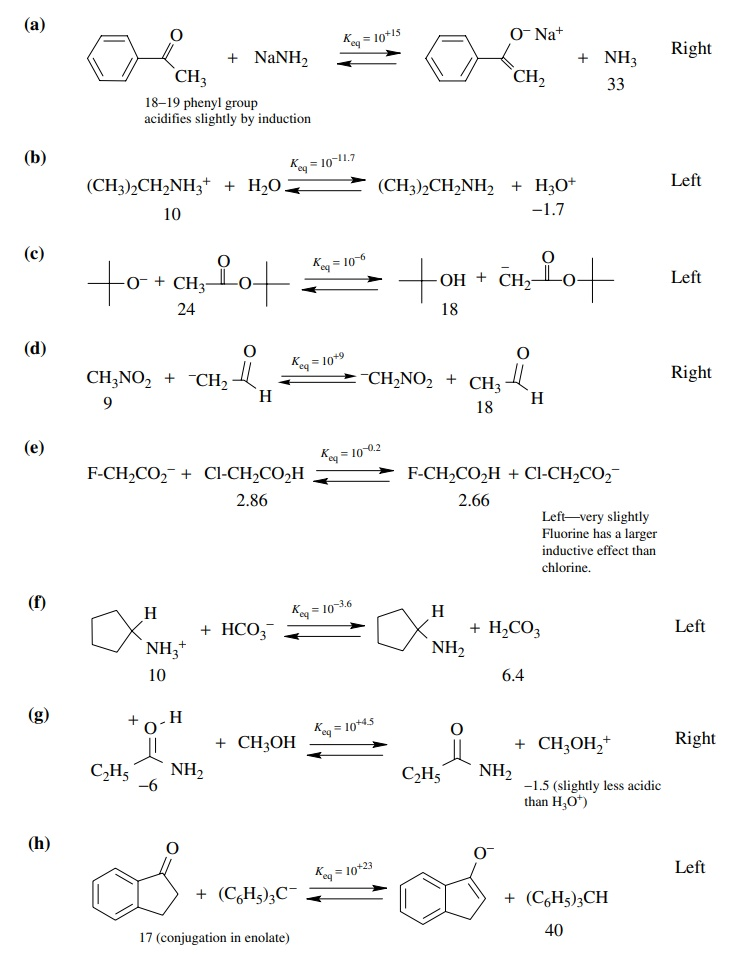3.3. List (by number) in order of increasing acidity of the underlined protons.(a) 1 < 4 < 2 < 3

(b) 2<1<3<4

(c) 4<1<2<3

(d) 4<2<3<1

(e) 1<4<3<2

(f) 4<2<3<1

(g) 1<4<3<2

(h) 3<2<1<4

(i) 1<3<2<4

(j) 4<1<2<3

(k) 4<2<3<1

3.4. Give explanations for the following observations:

(a) Amides are protonated on oxygen rather than on nitrogen.

(b) Ethers are better Lewis bases than ketones.

(c) Tetramethyl guanidine (pKa 12) is a much stronger base than N ,N - dimethylacetamide (pKa ≈ −0.5).(d) Boron trifluoride (BF3) is a stronger Lewis acid than trimethyl borate [(CH3O)3B].

(e) Piperidine is a much stronger Lewis base than pyridine.(f) p-Nitrophenol has pKa 8 whereas phenol has pKa 10.(g) o-Chloroaniline is a weaker base than p-chloroaniline.(h) Sodium borohydride in alcohol does not reduce imines effectively. If BF3 is added to the mixture, however, the reduction proceeds much more rapidly and efficiently.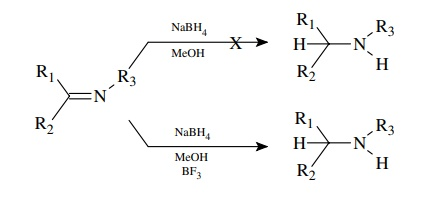(a) Amides are protonated on oxygen because a resonance-stabilized cation is produced. Protonation on nitrogen gives a localized cation.(b) The lone pairs on the ether oxygen are in sp3 while those of the ketone are in sp2 orbitals. Thus the e pairs of the ketone are more tightly bound and less able to be donated, making the ketone the weaker base.

(c) This is because the conjugate acid of tetramethylguanidine has much greater resonance stabilization than the N ,N -dimethyl amide (more forms and equiva-lent forms.)(d) The amount of e donation to boron by resonance is much greater for oxygen than it is for fluorine because of fluorine’s greater electronegativity. Therefore the boron of trimethyl borate is more e rich and it is a poorer Lewis acid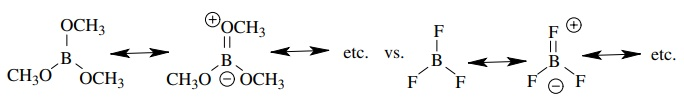(e) The lone pair of piperidine is in an sp3 orbital while the lone pair of pyridine is in an sp2 orbital. The greater s character causes the e to be more tightly bound and less able to be donated to H+ .

(f) The conjugate base of p-nitrophenol is resonance delocalized into the nitro group. The negative charge is thus more stable and the conjugate acid is more acidic.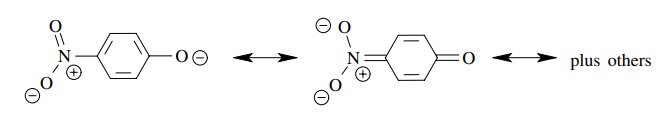(g) The inductive effect of the chlorine in the ortho position is greater than that of the para chloro group because it is closer to the amino group. The greater inductive effect removes more e density from nitrogen and makes the ortho isomer a weaker base.

(h) BF3 complexes with the lone pair of the imine and makes it much more electron deficient and thus electrophilic. In the complexed form it reacts with NaBH4 because it is a much better electrophile

3.5. Provide a rationale for the fact that hydrogen sulfide (H2S) is more acidic than water (H2O).Oxygen is more electronegative than sulfur so the hydroxide ion should be more stable than the hydrosulfide anion. This should result in water being more acidic. However, the hydrogen – sulfur bond is nearly 40 kcal/mol weaker than the hydro-gen – oxygen bond. Thus the energy cost of bond breaking for hydrogen sulfide is much less than for water. This overshadows the effect of electronegativity and makes hydrogen sulfide more acidic.

3.6. It has been found that benzenesulfonic acid (pKa ≈ −6) is a much stronger acid than benzoic acid (pKa 5). Discuss the factors that can account for this.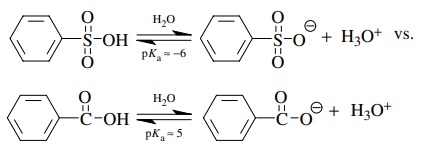Compare the conjugate bases. Since sulfur is slightly more electronegative than carbon and is bonded to two other oxygens rather than one, the inductive effect predicts benzenesulfonic acid should be stronger. But the huge difference in acidity is much more than can be explained by an inductive effect argument. The sulfonate ion has three equivalent resonance forms while the carboxylate group has only two. This could explain the difference. However, the sulfur in such systems is actually tetrahedral so any resonance argument must involve overlap with the d orbitals on sulfur. Another bonding picture is two coordinate covalent bonds from sulfur to oxygen. This leaves sulfur with a formal +2 charge right next to the anionic oxygen. Since these are merely a different electron, this could simply be considered an additional resonance form. Thus the greater resonance stabilization of the sulfonate anion makes the conjugate acid very acidic.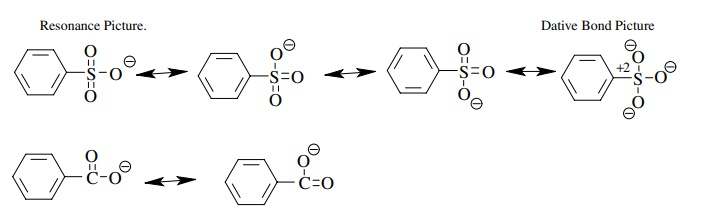3.7. The reaction of pyrrole with a strong acid leads to protonation on C-2, not on nitrogen. Explain why.Protonation at C-2 gives a resonance-stabilized (although nonaromatic) cation. Protonation at nitrogen destroys the aromaticity of the pyrrole ring by taking the lone pair out of the aromatic system. At the same time the cation produced is not resonance stabilized. Thus protonation on C-2 is favored.

3.8. By pKa measurements it can be shown that 1,1,1-bicyclopentanecarboxylic acid is 10 times more acidic than 2,2-dimethylpropanoic acid, even though both have a quaternary carbon attached to the carboxylic acid group. Account for this difference in pKa.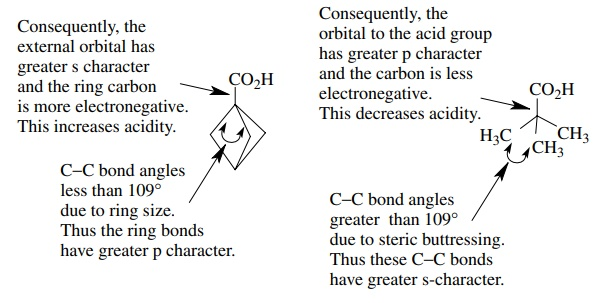3.9. It is observed that the rate of ionization of allylic benzoates is, in most cases, inversely proportional to the pKa’s of the corresponding benzoic acids. (i.e., the lower the pKa, the faster the rate of ionization.) Account for this correlation.